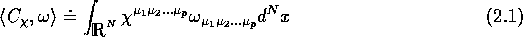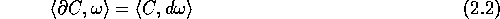Next: Grassmann algebras (a compendium Up: Differential forms over Grassmann Previous: Differential forms over Grassmann

## Classical analogies

From the algebraic point of view, it is convenient to consider the Grassmann algebra Gr(N) with N generators as the graded-commutative analogue of the commutative algebra generated by the coordinates . Clearly, (or better, A(N) at the algebraic level) is the simplest example of a manifold. In the same way, Gr(N) is the simplest example of a supermanifold.

In a course of elementary differential calculus, after having defined integration over , one builds the space of differential forms of degree p and extend the definition of the integral to the whole of . The space of differential forms over is built as the linear span (real coefficients) of products with . Then one proves a basic tool of calculus, namely the Stokes' theorem that relates the integral of a differential of the form over a particular subspace X to the integral of the form over the boundary of X. Finally, one proves the Poincaré Lemma: On , every closed (i.e. ) p-form, p>0 is exact (i.e. with ). Thus, De Rham cohomology of is trivial.

Physicists and mathematicians (also engineers!) know that, for many applications, one has to use distributions rather than functions. Usual distributions on are therefore well known and are properly defined as linear forms over an appropriate class of test functions. One can generalize this concept of distributions to forms. Such distributions are usually called De Rham currents, distributional forms or simply ``currents''  and are now a classical and necessary tool of analysis over (or over manifolds). In simple terms, a current is a linear machine that produces a number out of a p-form. A simple example of a p-current C can be obtained from any p-dimensional submanifold S (more properly from any p-chain) by writing . Completely antisymmetric contravariant tensors (so called ``p-vectors'') also define currents. Indeed, let denote the space of derivations on A(N); elements of are vector fields . Let us call the space of p-vectors, with elements As in the case of forms over A(N), this wedge product of p-vectors is antisymmetric. Such a p-vector defines a p-current: Let , one defines the current associated to via the equationOne can define a boundary operator mapping p-currents to p-1 as followsOf course, currents can be closed (i.e. ) or not. If we only consider ``regular'' objects, we can also associate p-vectors to currents and since we shall only stay at a purely formal level, i.e. forget about problems of analysis, this is what we shall do in most of the following and therefore identify p-currents and p-vectors. If denotes a p-vector with corresponding current C, we shall call , with components the (p-1)-vector associated with the current . We shall often use the same notation or C for both p-vectors and currents.

In classical differential calculus (commutative geometry), the next step is to generalize the above notions to the case of manifolds. As everybody knows, a new phenomenon appears: The Poincaré lemma may fail (a form that is closed is no longer necessarily exact!). However, our aim, in the present article, is to study (some) properties of Grassmann algebras related to currents and not to study the corresponding properties on arbitrary graded-commutative algebras. As stressed previously the former are simple graded-commutative analogues of : They are the simplest ``supermanifolds'' and, exactly as for , De Rham cohomology is trivial. We can therefore stop here this paragraph devoted to classical analogies.

The fact that the space of exterior forms over is isomorphic with Gr(N) is certainly true but can be confusing in the context of the present discussion. Let us repeat again that the analogy that we use here is between A(N) and Gr(N). We now turn our attention to the Grassmannian analogue of , the algebra of exterior forms over Gr(N).Next: Grassmann algebras (a compendium Up: Differential forms over Grassmann Previous: Differential forms over Grassmann

Robert Coquereaux
Mon May 20 14:40:14 MET DST 1996